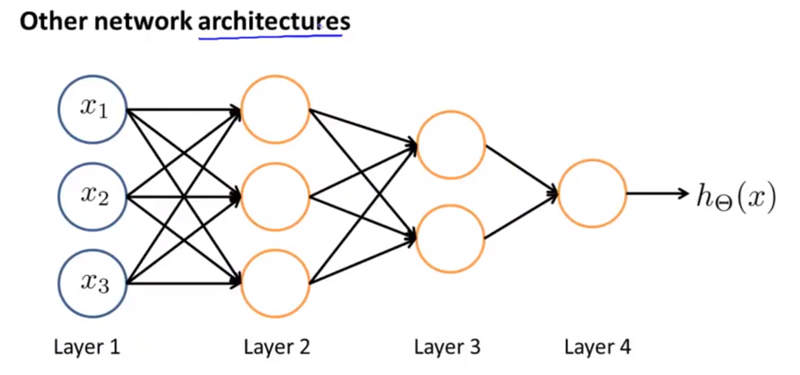## 非线性假设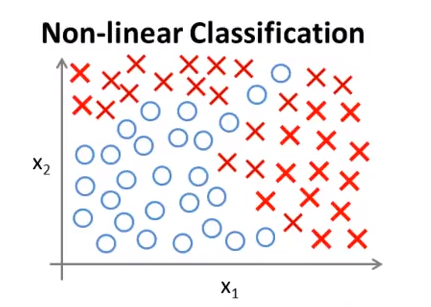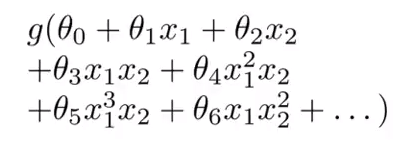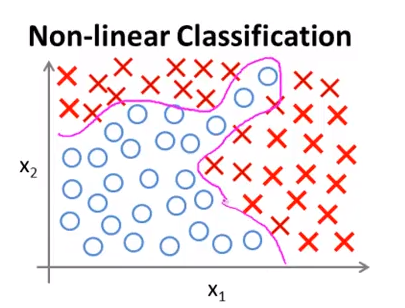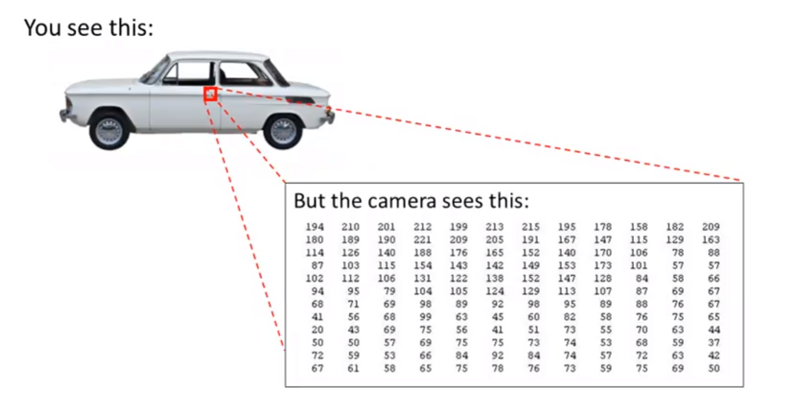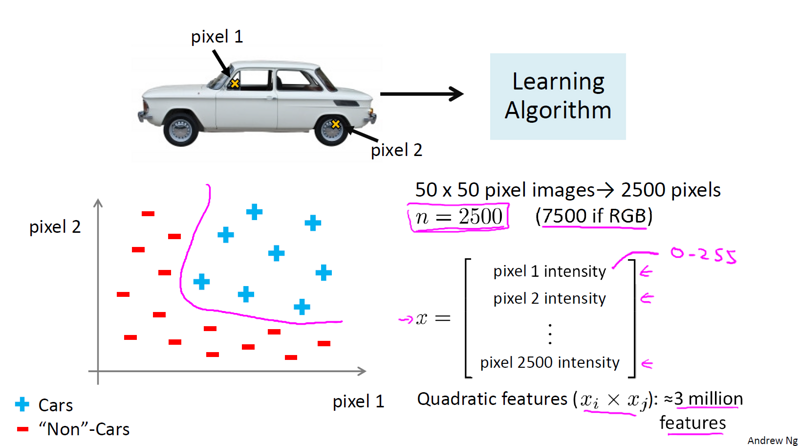## 神经元与大脑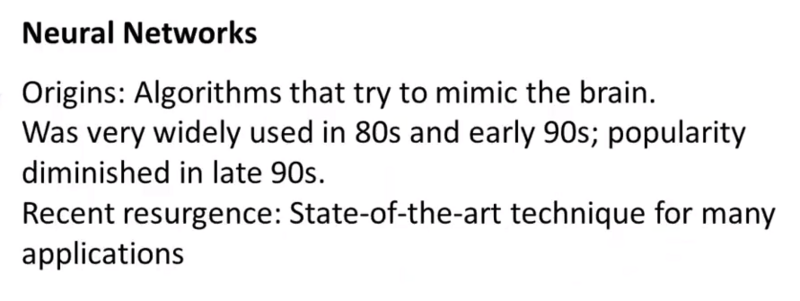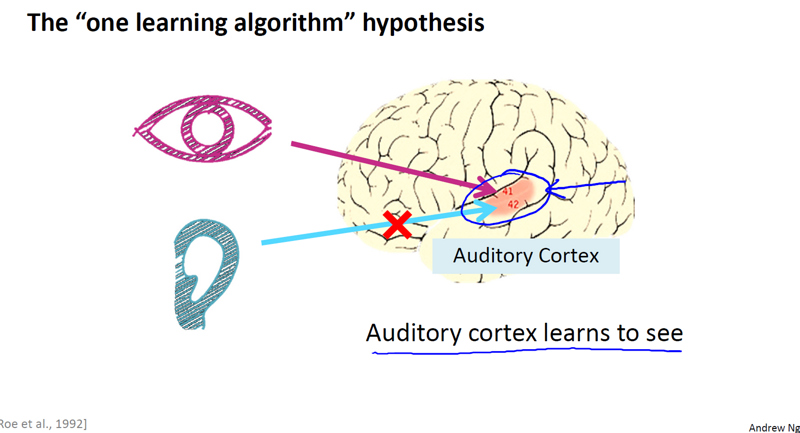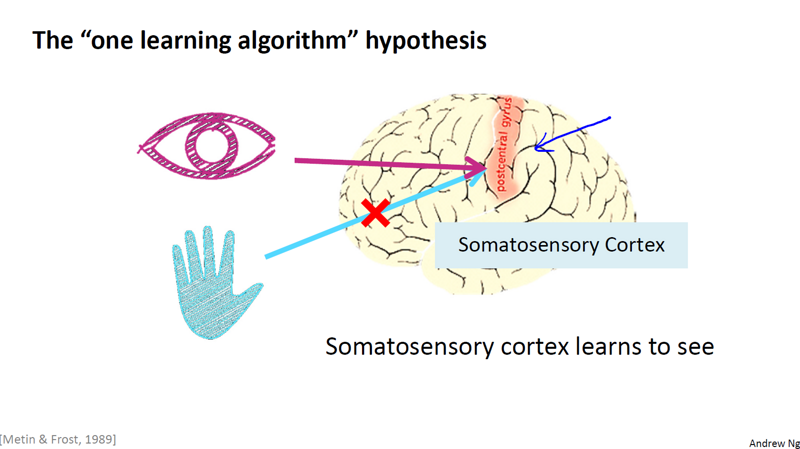## 模型展示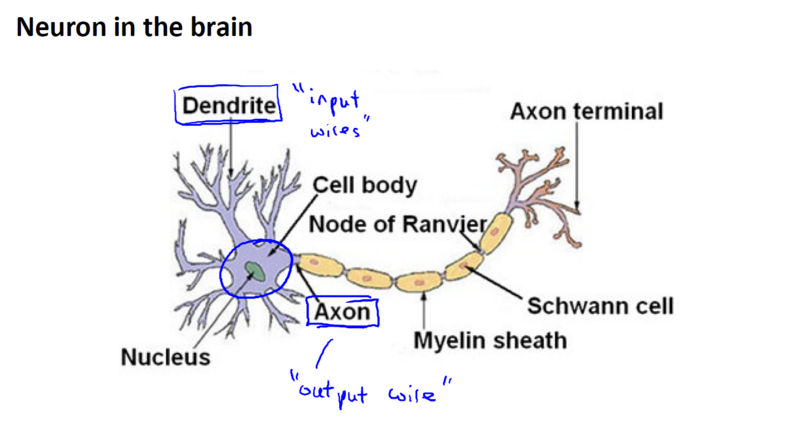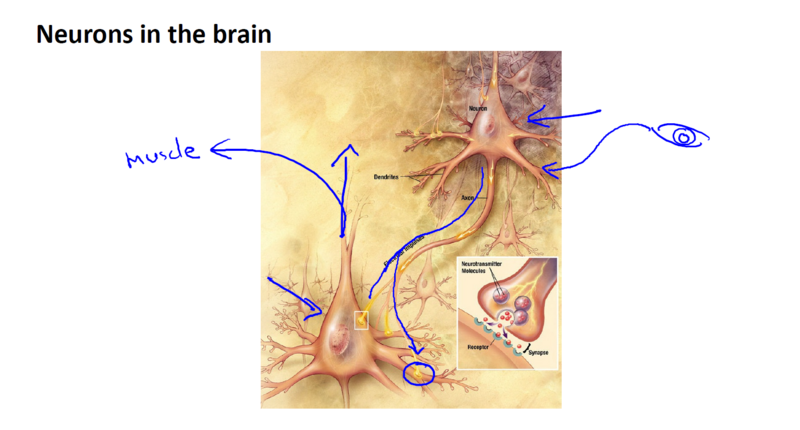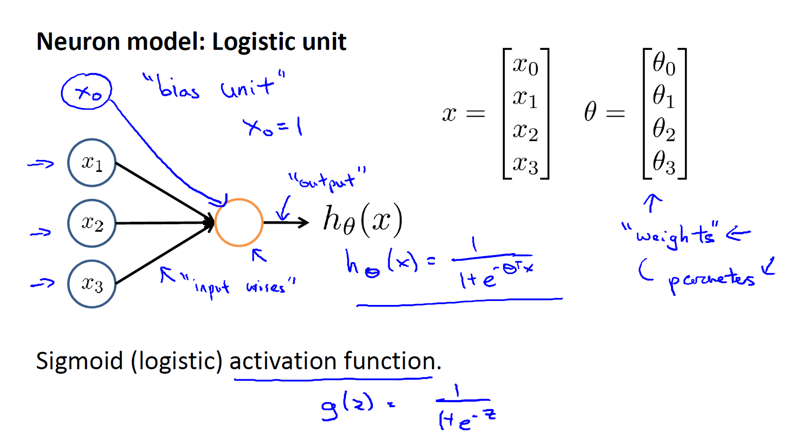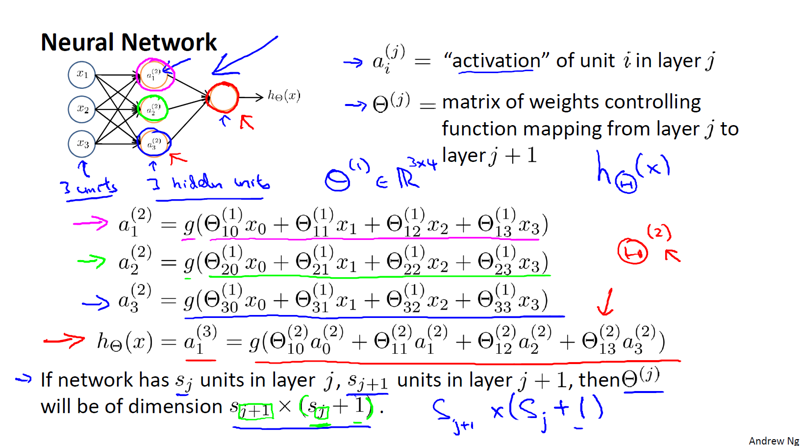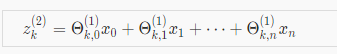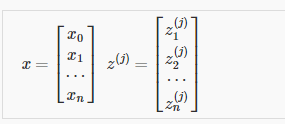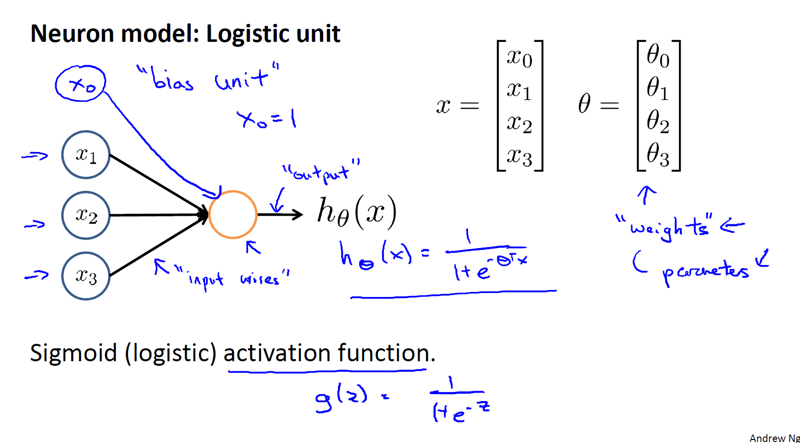z^(2) = \Theta^(1), a^(2) = g(z^(2)),这里的z^(2)是个三维向量，a^(2)也是个三维向量，因此这里的激励 g 将 sigmod 函数逐元素作用于z^(2)中的每个元素。这里我们还有隐藏的偏置单元只是未在图中画出，为了注意这额外的偏置单元，我们额外加上一个a_0^(2) = 1,这样a^(2)就是一个四维特征向量。然后我们为了计算假设的实际输出值，我们只需要计算z^(3),它等于上图中 g 括号中的部分，z^(3) = \Theta^(2)a^(2),则假设输出h_\Theta(x) = a^(3) = g(z^(3))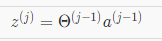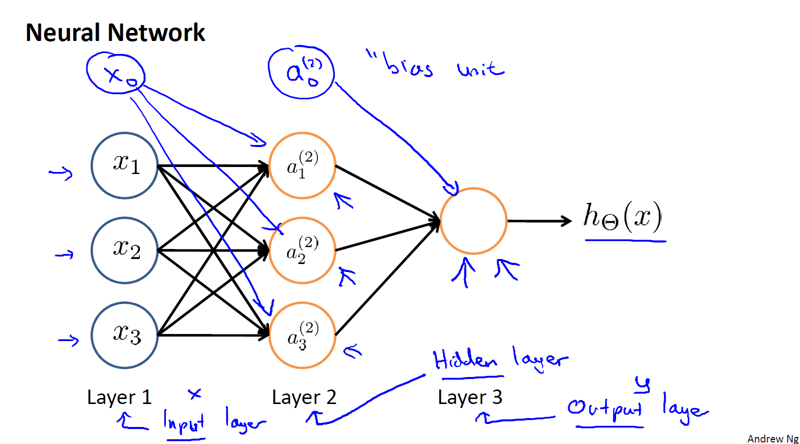h_\Theta(x) = g(\Theta_10^2a_0^2+\Theta_11^2a_1^2+\Theta_12^2a_2^2+\Theta_13^2a_3^2)